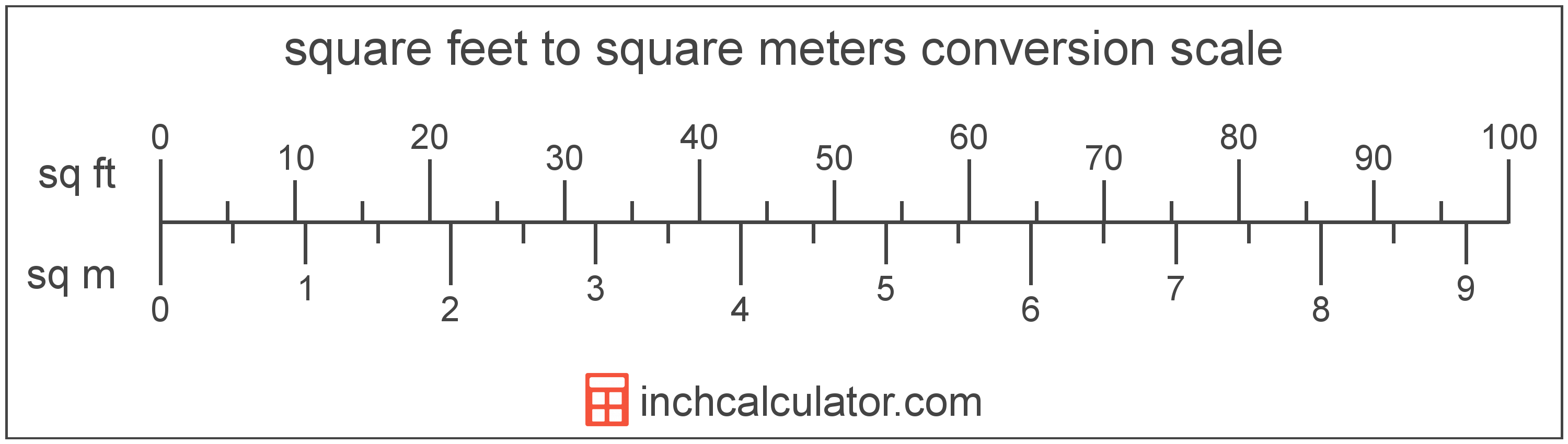# Square Meters to Square Feet Converter

Enter the area in square meters below to get the value converted to square feet.

(find square meters)
Results in Square Feet:1 sq m = 10.76391 sq ft

Do you want to convert square feet to square meters?

## How to Convert Square Meters to Square Feet

To convert a measurement in square meters to a measurement in square feet, multiply the area by the following conversion ratio: 10.76391 square feet/square meter.

Since one square meter is equal to 10.76391 square feet, you can use this simple formula to convert:

square feet = square meters × 10.76391

The area in square feet is equal to the area in square meters multiplied by 10.76391.

For example, here's how to convert 5 square meters to square feet using the formula above.
square feet = (5 sq m × 10.76391) = 53.819552 sq ft### How Many Square Feet Are in a Square Meter?

There are 10.76391 square feet in a square meter, which is why we use this value in the formula above.

1 sq m = 10.76391 sq ft

## What Is a Square Meter?

One square meter is equivalent to the area of a square with sides that are each 1 meter in length. One square meter is equal to 10.76391 square feet or 10,000 square centimeters.

The square meter, or square metre, is the SI derived unit for area in the metric system. A square meter is sometimes also referred to as a square m. Square meters can be abbreviated as sq m, and are also sometimes abbreviated as . For example, 1 square meter can be written as 1 sq m or 1 m².

You can use a square meters calculator to calculate the area of a space if you know its dimensions.

## What Is a Square Foot?

One square foot is equivalent to the area of a square with sides that are each 1 foot in length. One square foot is equal to 144 square inches or 0.092903 square meters .

The square foot is a US customary and imperial unit of area. A square foot is sometimes also referred to as a square ft. Square feet can be abbreviated as sq ft, and are also sometimes abbreviated as ft². For example, 1 square foot can be written as 1 sq ft or 1 ft².

You can use a square footage calculator to calculate the area of a space if you know its dimensions.

## Square Meter to Square Foot Conversion Table

Table showing various square meter measurements converted to square feet.
Square Meters Square Feet
1 sq m 10.76 sq ft
2 sq m 21.53 sq ft
3 sq m 32.29 sq ft
4 sq m 43.06 sq ft
5 sq m 53.82 sq ft
6 sq m 64.58 sq ft
7 sq m 75.35 sq ft
8 sq m 86.11 sq ft
9 sq m 96.88 sq ft
10 sq m 107.64 sq ft
11 sq m 118.4 sq ft
12 sq m 129.17 sq ft
13 sq m 139.93 sq ft
14 sq m 150.69 sq ft
15 sq m 161.46 sq ft
16 sq m 172.22 sq ft
17 sq m 182.99 sq ft
18 sq m 193.75 sq ft
19 sq m 204.51 sq ft
20 sq m 215.28 sq ft
21 sq m 226.04 sq ft
22 sq m 236.81 sq ft
23 sq m 247.57 sq ft
24 sq m 258.33 sq ft
25 sq m 269.1 sq ft
26 sq m 279.86 sq ft
27 sq m 290.63 sq ft
28 sq m 301.39 sq ft
29 sq m 312.15 sq ft
30 sq m 322.92 sq ft
31 sq m 333.68 sq ft
32 sq m 344.45 sq ft
33 sq m 355.21 sq ft
34 sq m 365.97 sq ft
35 sq m 376.74 sq ft
36 sq m 387.5 sq ft
37 sq m 398.26 sq ft
38 sq m 409.03 sq ft
39 sq m 419.79 sq ft
40 sq m 430.56 sq ft

## References

1. Collins Dictionary, Definition of 'square meter', https://www.collinsdictionary.com/us/dictionary/english/square-meter
2. Merriam-Webster, square foot, https://www.merriam-webster.com/dictionary/square%20foot